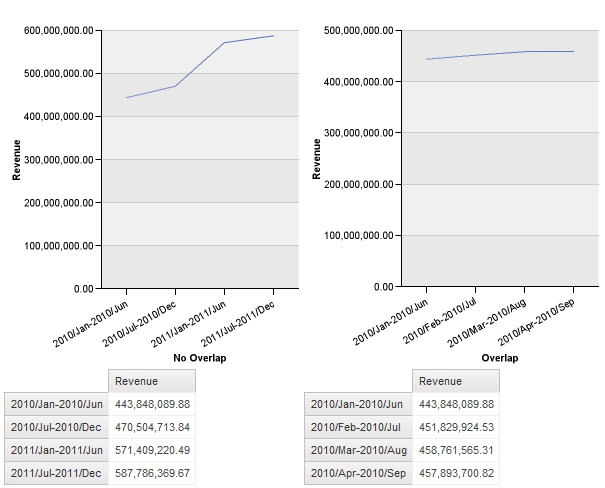# Dynamic Time Groups in an OLAP Cube

One of the challenges presented to me while I was helping out at the DHHS in New Hampshire was to find a way to make user defined year groups in the report. While it’s trivial to create year groupings in a cube, it becomes impossible when you need to account for every possible combination reports require. Sometimes you may need sets of 5 years starting at 1991. Other times the requirement may be every 2 years starting at 1980. Sometimes the users may want to see each year, 1991-1995, 1992-1996, 1993-1997; while other times the overlap is unnecessary, 1991-1995, 1996-2000, 2001-2006. Each measure would have to be aggregated for each grouping, increasing the size of the cube.

At first glance my solution is a bit complex. It uses JavaScript to control the appearance of the prompt and OLAP functions to set up the groups. Finally report expressions are used to control the labels in the chart and crosstab. Since the Cognos PowerCube samples have a limited set of years, I’ve adapted the technique to work on months instead.

In this example, the user is presented with three prompts. The first and second prompts allow the users to select the months they want. The first prompt has no overlap, if the group size selected is 6, it will show Jan-Jun and Jul-Dec. The second prompt will show Jan-Jun, Feb-Jul, Mar-Aug and so on. The third prompt shows the group size, defaulting to 6.The non-overlapping month prompt takes some work to get working. It is, essentially, filtering the month level where mod(monthNumber,groupSize) is 0. It is a little more complex, as that alone won’t work. First, the mod function isn’t supported by the cube, and will result in local processing. Second, it should be monthNumber – 1:

```(((total(
[One] within set periodsToDate(
[sales_and_marketing].[Time].[Time].[Time]
, currentMember([sales_and_marketing].[Time].[Time])
)
)-1)
/ #prompt('Group Size','integer','6')#)
-
floor((( total(
[One] within set periodsToDate(
[sales_and_marketing].[Time].[Time].[Time]
, currentMember([sales_and_marketing].[Time].[Time])
)
)-1)
/ #prompt('Group Size','integer','6')#)))
* #prompt('Group Size','integer','6')#
```

If you want the group to have a different start month, change the -1.

That will give us a set of every sixth month. 2010/Jan, 2010/Feb, 2011/Jan, 2011/Feb. But now we need to modify the appearance of the prompt values. For that we need JavaScript.

This JS will loop through the prompt, convert the month name into a numeric value, add the selected group size, then convert that number back into a month and concatenate it onto the label again.

```<script>
var fW = (typeof getFormWarpRequest == "function" ? getFormWarpRequest() : document.forms["formWarpRequest"]);
if ( !fW || fW == undefined)
{ fW = ( formWarpRequest_THIS_ ? formWarpRequest_THIS_ : formWarpRequest_NS_ );}

function parseMonthName(name) {
var Months = ['Jan','Feb','Mar','Apr','May','Jun','Jul','Aug','Sep','Oct','Nov','Dec'];
for (i=0;i<12;i++){ if(Months[i]==name) {return i+1; break;}}
}

month = (month + add)%12;
month=month==0?12:month;
return month;
}

function getMonthName(month) {
var Months = ['Jan','Feb','Mar','Apr','May','Jun','Jul','Aug','Sep','Oct','Nov','Dec'];
return Months[month-1];
}

function setMonthNames(startPrompt,endPrompt){

var Size
, Start = startPrompt
, End = endPrompt
, Month , newMonth, newYear

// Loop through the groupSize list to set the size variable. Subtract 1 from the value since the value includes the current month.
for(var i =0;i<fW._oLstChoices_groupSize.length;i++){if(fW._oLstChoices_groupSize[i].selected) Size=fW._oLstChoices_groupSize[i].value -1}

for (var i = 0;i<Start.length;i++){
if(!Start[i].getAttribute('Orig')) {Start[i].setAttribute('Orig' , Start[i].getAttribute('dv')); };
Month = Start[i].getAttribute('Orig');

// If the group size is set to 0, use the original label. This is for on the fly changes.
if(Size==0) {
Start[i].setAttribute('dv' , Month);
}
else {
newMonth = parseMonthName(Month.substr(5,3)) ;
Start[i].setAttribute('dv' , Month + '-' +  newYear + '/' + getMonthName(addMonths(newMonth,Size)));
}
Start[i].innerHTML= Start[i].getAttribute('dv') ;
//if(i>=Size-1) {Start[i].display='none';} else {Start[i].display='';} //Really, IE? Really?!
}
}
</script>
```

So far this has only served to make it easier for the end user to understand how he’s filtering his report. The prompts themselves are still passing single member unique names to the query. Another calculated data item will be needed to group the years.

```member(
total(
currentMeasure
within set
lastPeriods(
0-#prompt('Group Size','integer','5')#
,currentMember([sales_and_marketing].[Time].[Time])
)
)
,'Grouped Total'
,'Grouped Total'
,[sales_and_marketing].[Time].[Time])
```

The lastPeriods will return the next Group Size members on the same level. This calculated member can then be used as a tuple on any measure that needs to be grouped. In the attached report I simply added it as the default measure in the charts and crosstabs.

That will create the groupings, but the labels on the chart and crosstab will still only show a single month. To fix that we can use a report expression with the same logic as the JavaScript from the prompt page:

```case
when ParamValue('Group Size') = '1' then [Report].[No Overlap]
else
[Report].[No Overlap]  + '-'
+ number2string(
string2int32(substring([Report].[No Overlap],1,4))
+ case
when mod (
case substring([Report].[No Overlap],6,3)
when 'Jan' then 1
when 'Feb' then 2
when 'Mar' then 3
when 'Apr' then 4
when 'May' then 5
when 'Jun' then 6
when 'Jul' then 7
when 'Aug' then 8
when 'Sep' then 9
when 'Oct' then 10
when 'Nov' then 11
when 'Dec' then 12
end
+ string2int32(ParamValue('Group Size'))-1,12) between 1 and (string2int32(ParamValue('Group Size'))-1)
then 1
else 0
end
)
+'/'+
case mod (
case substring([Report].[No Overlap],6,3)
when 'Jan' then 1
when 'Feb' then 2
when 'Mar' then 3
when 'Apr' then 4
when 'May' then 5
when 'Jun' then 6
when 'Jul' then 7
when 'Aug' then 8
when 'Sep' then 9
when 'Oct' then 10
when 'Nov' then 11
when 'Dec' then 12
end + string2int32(ParamValue('Group Size'))-1,12)
when 1 then 'Jan'
when 2 then 'Feb'
when 3 then 'Mar'
when 4 then 'Apr'
when 5 then 'May'
when 6 then 'Jun'
when 7 then 'Jul'
when 8 then 'Aug'
when 9 then 'Sep'
when 10 then 'Nov'
when 11 then 'Oct'
when 0 then 'Dec'
end
end```

Simply change the text source on the crosstab or chart node member to Report Expression and paste that in.Report XML